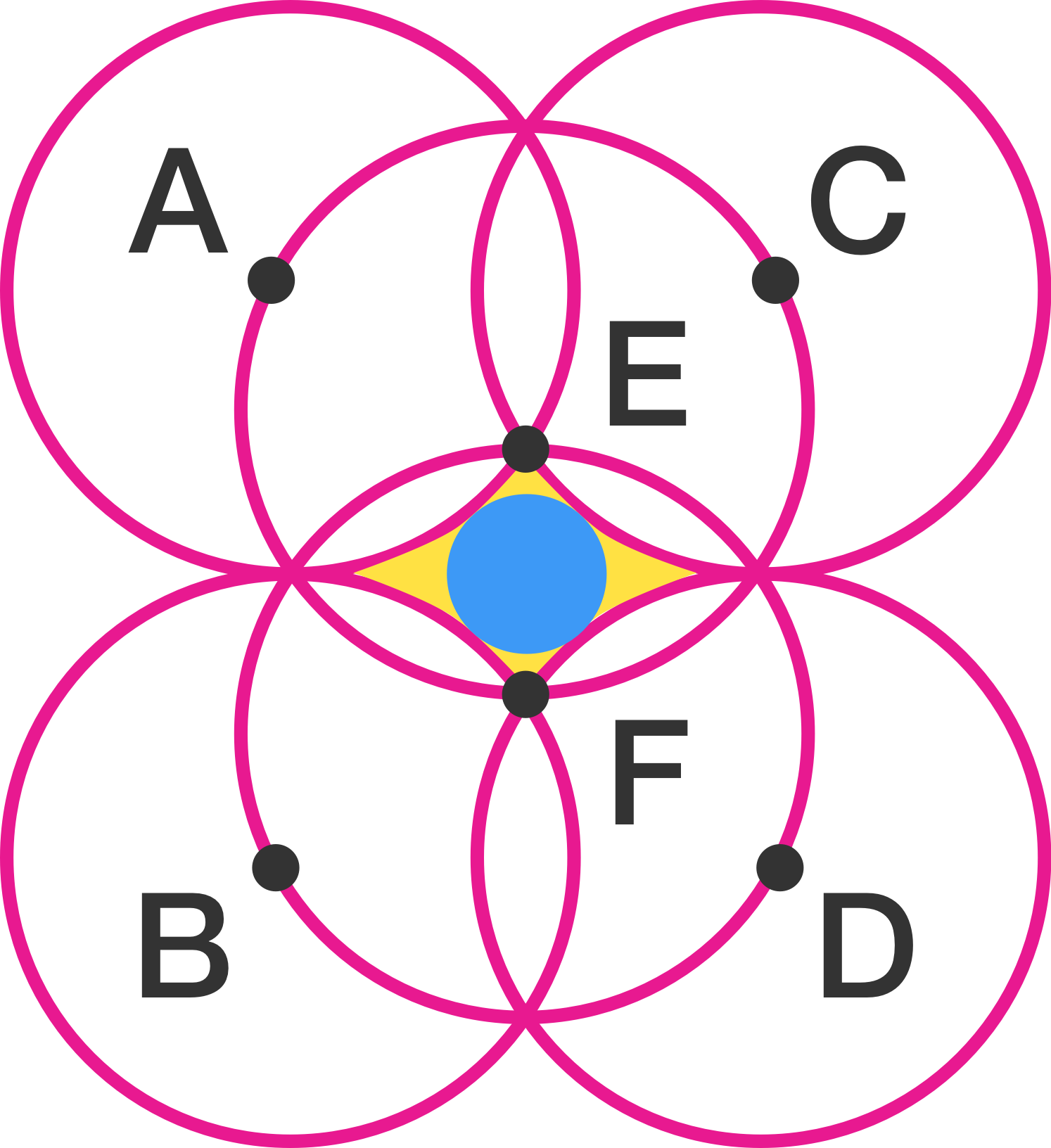The Gemstone

Geometry Level 3If all circles with centers $A, B, C, D, E$ and $F$ have the same radius of 7, what is the area of the blue circle squeezed into the yellow shaded region given that circles $E$ and $F$ passes through the centers of the other circles.

Give your answer to 2 decimal places.

×